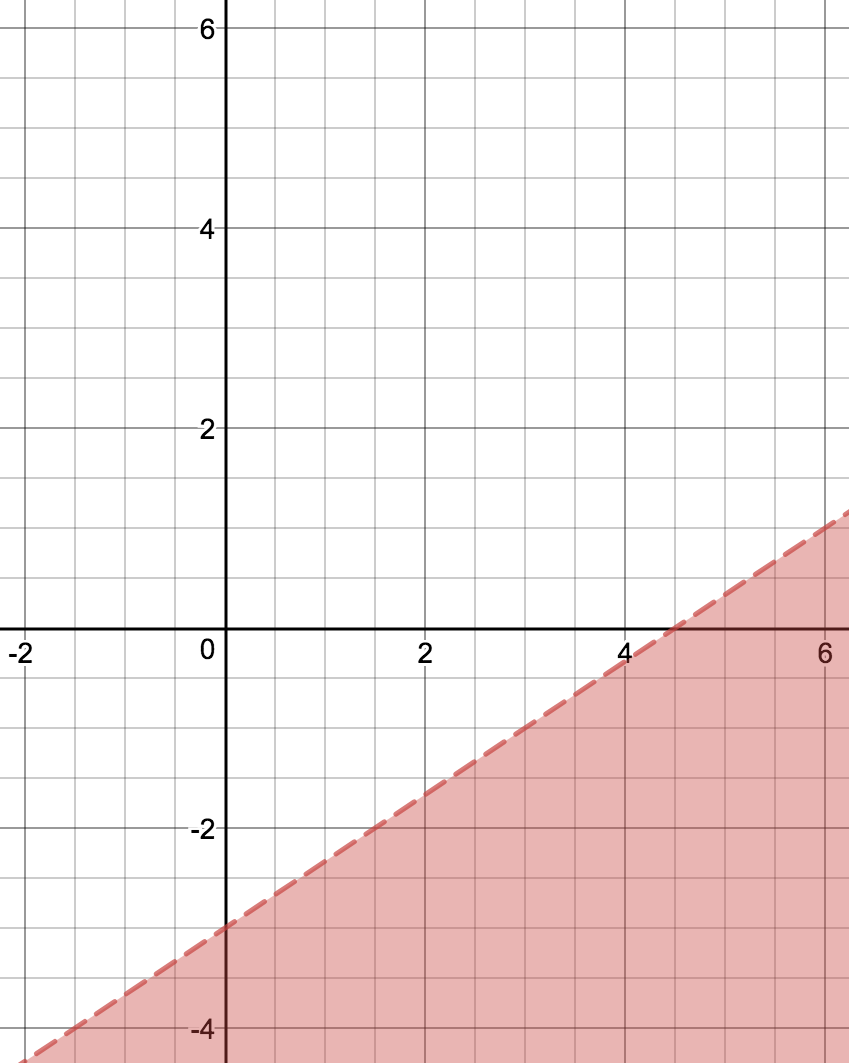# Linear Equations, Inequalities and Systems

## Objective

Describe the solutions and features of a linear inequality. Graph linear inequalities.

## Common Core Standards

### Core Standards

?

• A.REI.D.12 — Graph the solutions to a linear inequality in two variables as a half-plane (excluding the boundary in the case of a strict inequality), and graph the solution set to a system of linear inequalities in two variables as the intersection of the corresponding half-planes.

?

• 7.EE.B.4.B

• 8.EE.B.5

• 8.EE.B.6

## Criteria for Success

?

1. Given a linear equation, identify how the solution set will change when the equal sign is changed to a less than, less than or equal to, greater than, or greater than or equal to sign.
2. Match graphs of linear inequalities to algebraic representations of linear inequalities by testing points and analyzing the inequality.
3. Graph a linear inequality by identifying the features of the linear equation and then modifying the solution set to represent an inequality.

## Tips for Teachers

?

This lesson is the first in a series of three on linear inequalities. Students studied one-variable inequalities in grade 8, as well as graphing and solving linear equations, but this is the first time they have graphed, written, or identified solutions for linear inequalities.

## Anchor Problems

?

### Problem 1

Plot three points on the graph so that the value of $y$ in each ordered pair is greater than the value of $x$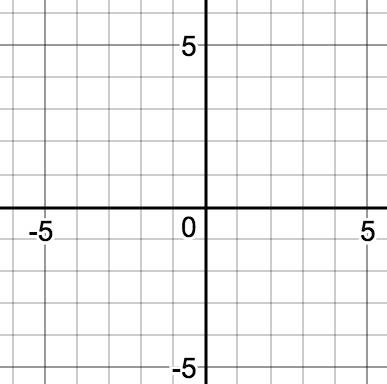#### References

Student Screen 2

Introduction to Linear Inequalities by Bob Lochel is made available by Desmos. Copyright © 2017 Desmos, Inc. Accessed Oct. 20, 2017, 4:04 p.m..

Modified by The Match Foundation, Inc.

### Problem 2

Below is the graph of the inequality ${y > 2x+1}$.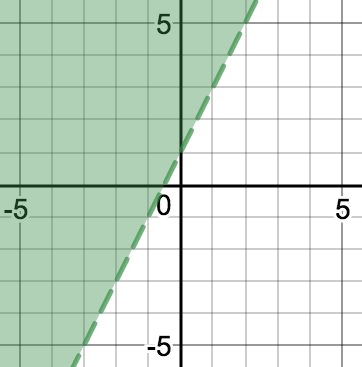• Name a point that is a solution. Explain your reasoning using the graph and algebraically.
• Name a point that is not a solution. Explain your reasoning using the graph and algebraically.

### Problem 3

Graph the following inequalities shown below:

 ${x+y\leq 20}$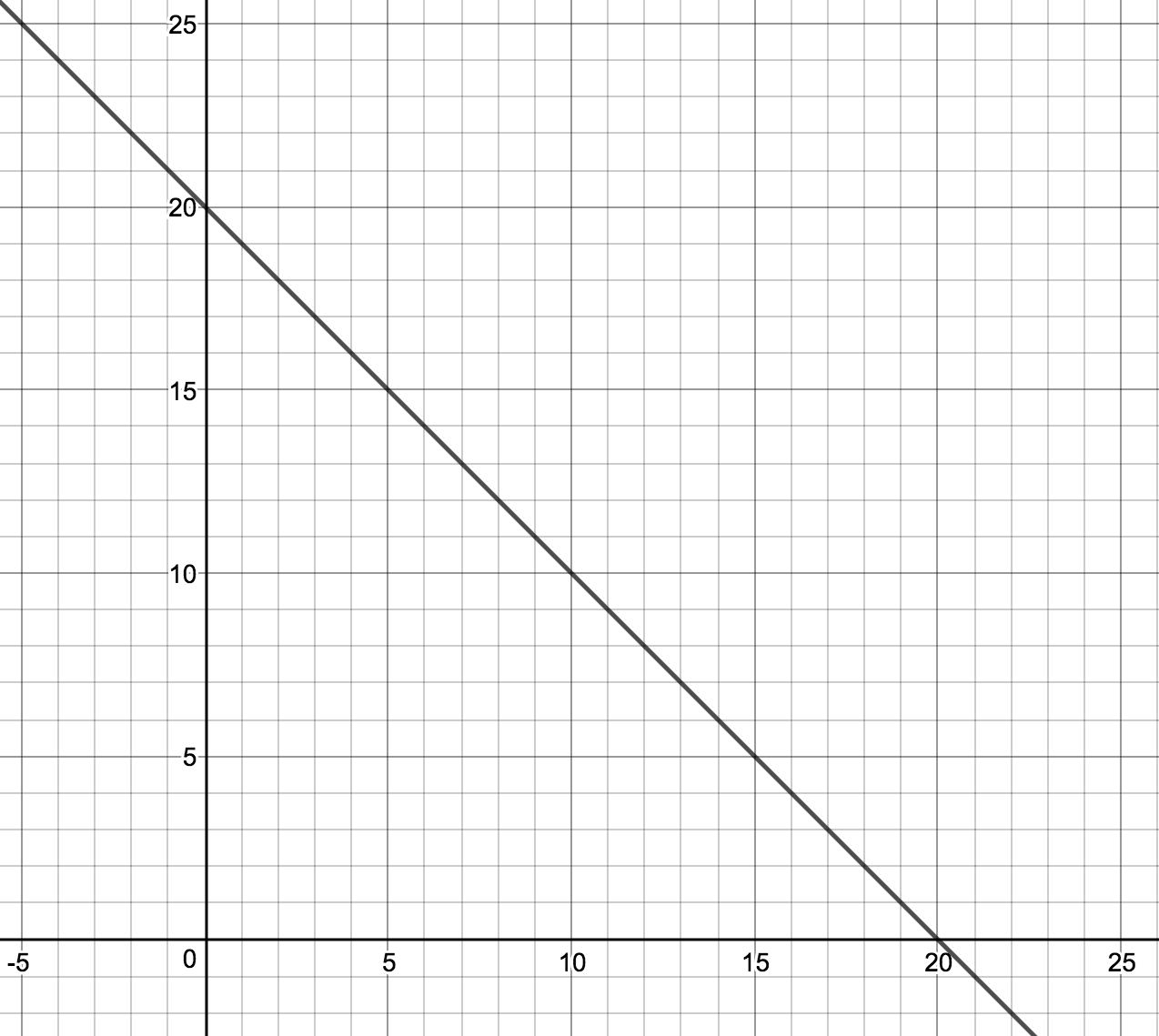${x+y< 20}$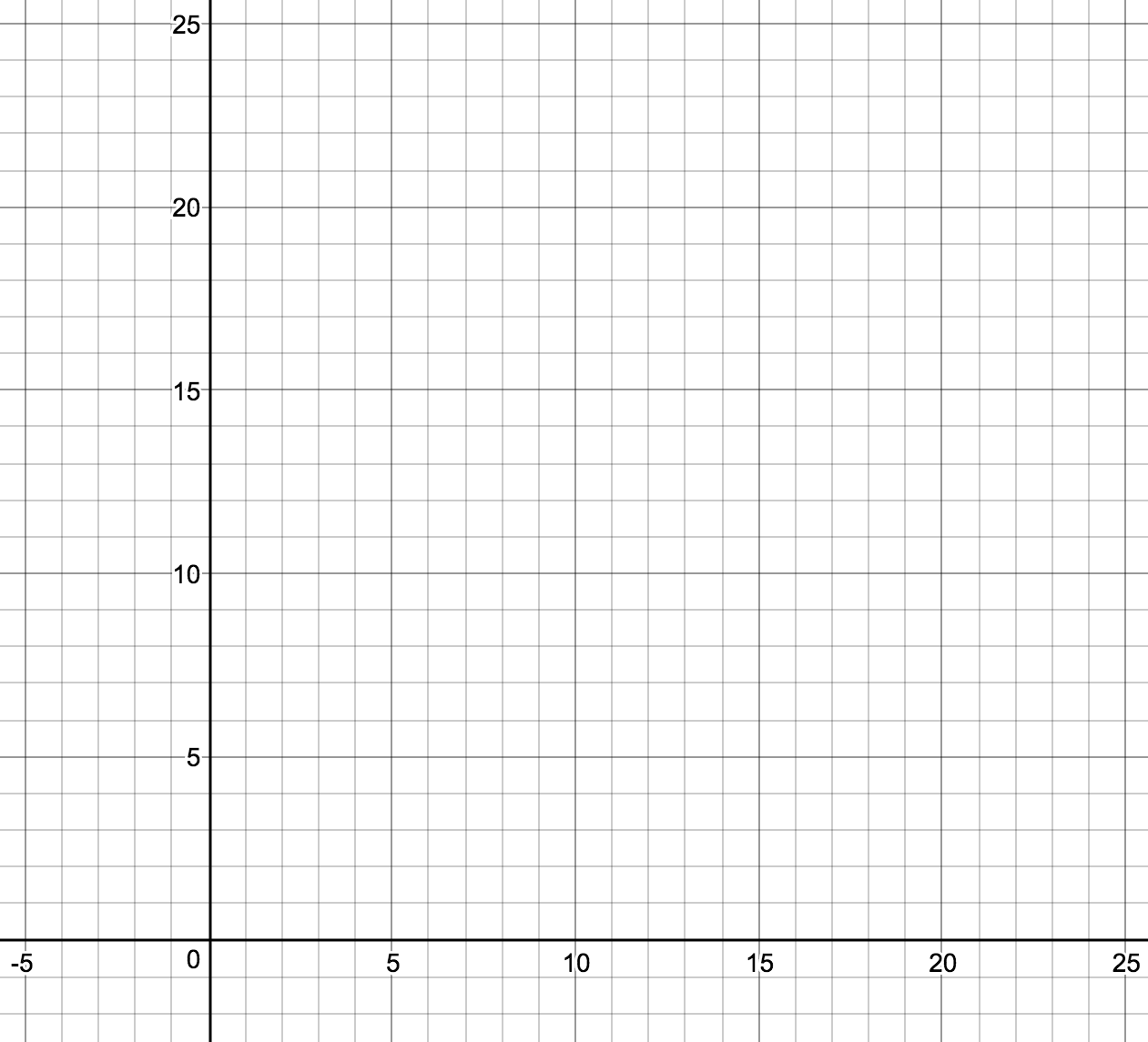${x+y\geq 20}$${x+y> 20}$#### References

EngageNY Mathematics Algebra I > Module 1 > Topic C > Lesson 21Example 1

Algebra I > Module 1 > Topic C > Lesson 21 of the New York State Common Core Mathematics Curriculum from EngageNY and Great Minds. © 2015 Great Minds. Licensed by EngageNY of the New York State Education Department under the CC BY-NC-SA 3.0 US license. Accessed Dec. 2, 2016, 5:15 p.m..

Modified by The Match Foundation, Inc.

## Problem Set

?

The following resources include problems and activities aligned to the objective of the lesson that can be used to create your own problem set.

• Include problems structured like “Which One Doesn’t Belong?”  highlighting the similarities and differences between graphs.
• Include problems where students are given a set of solutions and need to graph more than one inequality that will include these points as solutions.
• Include error analysis problems using the features of a linear inequality.

${2x-3y\leq 9}$